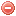# Description

The National fire danger rating system (NFDRS) was developed in the USA in the late 1960s and become operational in 1972. The NFDRS was updated in 1977 (cf. Burgan et al. 1977, Deeming et al. 1977), and later revised in order to better perform in the humid conditions prevailing in the Eastern United States (Burgan et al. 1988).

The NFDRS assesses fire danger by evaluating the upper limit of the behavior of potential fires in the rating area, and is intended to provide guidance for the planning of pre-suppression and suppression measures (Deeming et al. 1977).

The NFDRS is composed of four indices: the Lightning-caused fire occurrence index, the Man-caused fire occurrence index, the Burning index, and the (final) Fire load index. These indices are derived from fire behavior components (ignition, spread and energy release components) and risks (lightning and man-caused), which are themselves calculated based on meteorological observations, fuel moisture models, fuel models and topographical data (Deeming et al. 1977).

Only dead fuel moisture models (1-hour, 10-hour, 100-hour and 1000-hour timelags) will be addressed here, as they simply require meteorological data as input variables. The dead fuel moisture models for 100-hour and 1000-hour timelags will also be considered here, although they require precipitation duration as input variable, which is not a standard available meteorological variable.

The meteorological data used for the calculation of the NFDRS have to be recorded at early to mid-afternoon time (1 to 3 pm).

# References:

Original publication:
Deeming et al. (1977)

Other publications:
Burgan et al. (1977)
Burgan et al. (1988)

### Bibliography SearchNo results!

No results were found. Are you sure you searched for a tag?

### Symbols

 Variable Description Unit $$T$$ air temperature °C $$T_{dew}$$ dew point temperature °C $$H$$ air humidity % $$P$$ rainfall mm $$U$$ windspeed m/s $$w$$ days since last rain (or rain above threshold) d $$rr$$ days with consecutive rain d $$\Delta t$$ time increment d $$\Delta{e}$$ vapor pressure deficit kPa $$e_s$$ saturation vapor pressure kPa $$e_a$$ actual vapor pressure kPa $$p_{atm}$$ atmospheric pressure kPa $$PET$$ potential evapotranspiration mm/d $$r$$ soil water reserve mm $$r_s$$ surface water reserve mm $$EMC$$ equilibrium moisture content % $$DF$$ drought factor - $$N$$ daylight hours hr $$D$$ weighted 24-hr average moisture condition hr $$\omega$$ sunset hour angle rad $$\delta$$ solar declination rad $$\varphi$$ latitude rad $$Cc$$ cloud cover Okta $$J$$ day of the year (1..365/366) - $$I$$ heat index - $$R_n$$ net radiation MJ⋅m-2⋅d-1 $$R_a$$ daily extraterrestrial radiation MJ⋅m-2⋅d-1 $$R_s$$ solar radiation MJ⋅m-2⋅d-1 $$R_{so}$$ clear-sky solar radiation MJ⋅m-2⋅d-1 $$R_{ns}$$ net shortwave radiation MJ⋅m-2⋅d-1 $$R_{nl}$$ net longwave radiation MJ⋅m-2⋅d-1 $$\lambda$$ latent heat of vaporization MJ/kg $$z$$ elevation m a.s.l. $$d_r$$ inverse relative distance Earth-Sun - $$\alpha$$ albedo or canopy reflection coefficient - $$\Delta$$ slope of the saturation vapor pressure curve kPa/°C $$Cc$$ cloud cover eights $$ROS$$ rate of spread m/h $$RSF$$ rate of spread factor - $$WF$$ wind factor - $$WRF$$ water reserve factor - $$FH$$ false relative humidity - $$FAF$$ fuel availability factor - $$PC$$ phenological coefficient -

 Suffix Description $$-$$ mean / daily value $$_{max}$$ maximum value $$_{min}$$ minimum value $$_{12}$$ value at 12:00 $$_{13}$$ value at 13:00 $$_{15}$$ value at 15:00 $$_{m}$$ montly value $$_{y}$$ yearly value $$_{f/a}$$ value at fuel-atmosphere interface $$_{dur}$$ duration $$_{soil}$$ value at soil level

 Constant Description $$e$$ Euler's number $$\gamma$$ psychrometric constant $$G_{SC}$$ solar constant $$\sigma$$ Stefan-Bolzmann constant